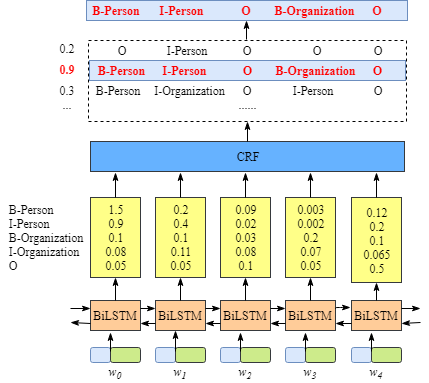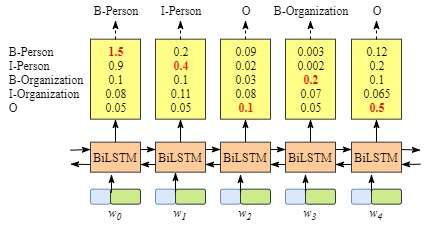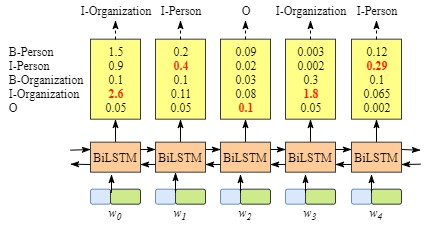circlepi's blog Even with an intractable probelm, one can find a way to do the right thing. 2019-08-14T15:19:59.846Z http://state-of-art.top/ circlepi Hexo 软性上下文数据增强技术（Soft Contextual Data Augmentation, SCA） http://state-of-art.top/2019/08/14/软性上下文增强技术/ 2019-08-14T15:20:08.000Z 2019-08-14T15:19:59.846Z 软性上下文数据增强(Soft Contextual Data Augmentation, SCA)

## 常见的文本数据增强技术

• Swap：随机交换相邻位置词（字）的位置，判断两个词是否相邻，可自定义一个大小为k的窗口，位置距离在k以内，则相邻，反之，则不相邻
• Dropout：以一定概率随机删除词（字）
• Smooth：以一定概率将词（字）替换成按照频率分布从词典中采样得到的词（字）
• 同义词替换
• LM_sample：以一定概率将词（字）替换成按照语言模型分布从词典中采样得到的词（字）
• BackTranslation: 回译，常用于机器翻译中

## SCA

$$P(w)=(p_1(w), p_2(w), …, p_{|V|(w)}$$

$$e_w = P(w)E=\Sigma^{|V|}_{j=0}p_j(w)E_j$$

## 实现

SCA架构如图1所示。具体怎么实现呢？• 先分别基于source和target平行语料训练两个语言模型，是自回归还是自编码都可以，论文中采用的是Tarnsformer，这样embedding就有了
• 正常进行NMT任务。对于要替换的词，用语言模型先来预测一遍，得到概率分布，和embedding做个计算就能得到soft word embedding了，实现起来改下embedding的加载就可以了。
]]>
Configargparser Usage http://state-of-art.top/2019/02/03/Configargparser usage/ 2019-02-03T15:30:08.000Z 2019-02-03T14:02:04.510Z How to Use Configargparser

## Introduction

In this blog, I want to show you somethings about how to use the “Configargparser” module to realize some basic config for your procedures. Configargparser is a more stronger module, but the relevant document is very few.

There are three main ways that programs get data from their environment when they start up:

• Commandline arguments,
• Configuration files
• Environment variables

Currently, if a python program wants to parse data from these sources, it needs to first check the environment variables, then check for a configuration file and finally look at the commandline arguments. The general cascade is that commandline arguments should override config files which should override environment variables. Unfortunately, checking all of these places is a major pain – which is what configargparser is meant to address.

It’s known that Python has provided two modules, argparser for commandline arguments parsing and configparser for configuration files. While Configparser can address those in a single module.

## Install

If Internet is available, you can install Configargparse with:

https://pypi.org/project/ConfigArgParse/

and then install from source by:

By the way, the repo on Github is also available at:

https://github.com/mgilson/configargparser

## Basic Usage

If you are similar with argparse, you can skip this section, because configargparser use the same way as argparser.

Run the file by:

You can see:

## From Config File

We can also load the config parameters from config file, a .ini file or .yml file. For example, we first prepare a test.yml file with a key: value format:

Run the file by:

You can see:

Run the file by:

You can see:

This means that the command line arguments will overwrite the config file arguments.

Some tips:

• The parameters in the yml file adapt the format as key: value. Note the whitespace after :

• When reading from a config file, you need also add the arguments to the parser by:

## Group

For clarity, we usually use argument group for different codes.

Run the file by:

You will see:

]]>
Transformer原理和实现 从入门到精通 http://state-of-art.top/2019/01/17/Transformer原理和实现-从入门到精通/ 2019-01-17T15:20:08.000Z 2019-01-17T12:48:10.451Z Transformer原理和实现 从入门到精通

## 概述

• 掌握Encoder-Decoder框架
• 掌握残差网络
• 掌握BatchNormalization(批归一化)和LayerNormalization（层归一化）
• 掌握Position Embedding（位置编码）

Notes: 本文代码参考哈弗大学的The Annotated Transformer

## Encoder-Decoder框架

Encoder-Decoder是为seq2seq（序列到序列）量身打造的一个深度学习框架，在机器翻译、机器问答等领域有着广泛的应用。这是一个抽象的框架，由两个组件：Encoder（编码器）和Decoder(解码器)组成。对于给定的输入source（x1, x2, x3, …,xn）, 首先编码器将其编码成一个中间表示向量z=（z1, z2, …, zn）。接着，解码器根据z和解码器自身前面的输出，来生成下一个单词（如Figure 1所示）。Figure 1

## 整体架构Figure 2

## Encoder

### EncoderLayer和残差网络

EncoderLayer如Figure 3所示。Figure 3Figure 4

• 输入x
• x做一个层归一化： x1 = norm(x)
• 进入多头self-attention: x2 = self_attn(x1)
• 残差加成：x3 = x + x2
• 再做个层归一化：x4 = norm(x3)
• 经过前馈网络: x5 = feed_forward(x4)
• 残差加成: x6 = x3 + x5
• 输出x6

### 多头注意力机制### 层归一化

#### BatchNormalization

$$z = g（Wu+b）$$

$$x=Wu+b$$

$$z = sigmoid(x) = g(x) = \frac{1}{1+exp(-x)}$$

$$\frac{\partial z}{\partial W} = \frac{\partial z}{\partial g}\cdot\frac{\partial g}{\partial x}\cdot\frac{\partial x}{\partial u}$$Figure 5

BatchNormalization就是解决这两个问题的。首先，它将隐藏层的输入强行变换为同一分布（解决了第一个问题），这个分布就是正态分布（解决了第二个问题）。Figure 6

#### LayerNormalization

BatchNormalization简直是个救世主啊，它令调参工作变得从未如此容易，让调参侠们不费吹灰之力，谈笑间到达收敛的彼岸。但毛主席曾经说过，万物都是辩证的，它同样存在两个问题：

• 对batch_size非常敏感。BatchNormalization的一个重要出发点是保持每层输入的数据同分布。回想下开始那个独立同分布的假设。假如取的batch_size很小，那显然有些Mini-Batch的数据分布就很可能与整个数据集的分布不一致了，又出现了那个问题，数据分布不一致…这就等于说没起到同分布的作用了，或者说同分布得不充分。实验也证明，batch_size取得大一点， 数据shuffle的好一点，BatchNormalization的效果就越好。
• 不能很方便地用于RNN。这其实是第一个问题的引申。我们再来看一下Figure 6中的均值和方差的计算公式。对所有样本求均值。对于图片这类等长的输入来说，这很容易操作，在每个维度加加除除就可以了，因为维度总是一致的。而对于不等长的文本来说，RNN中的每个time step共享了同一组权重。在应用BatchNormalization时，这就要求对每个time step的batch_size个输入计算一个均值和方差。那么问题就来了，假如有一个句子S非常长，那就意味着对S而言，总会有个time_step的batch_size为1，均值方差没意义，这就导致了BatchNormalization在RNN上无用武之地了。

LayerNormalization的主要变化在于：

• 不再对Mini-Batch中的N的样本在各个维度做归一化，而是针对同一层的所有神经元做归一化。归一化公式为：
$$\mu^l = \frac{1}{H}\Sigma_1^Ha_i^l$$

$$\sigma^l = \sqrt{\frac{1}{H}\Sigma_1^H(a_i^l-\mu^l)}$$

其中，H指的是一层神经网络的神经元个数。我们再回想下BatchNormalization，其实它是在每个神经元上对batch_size个数据做归一化，每个神经元的均值和方差均不相同。而LayerNormalization则是对所有神经元做一个归一化，这就跟batch_size无关了。哪怕batch_size为1，这里的均值和方差只和神经元的个数有关系（如果读到这里仍然感到不是特别清楚，再读两遍…还困惑也没关系，待会看Figure 7）。

• 测试的时候可以直接利用LN，所以训练时不用保存均值和方差，这节省了内存空间。

Figure 7示意了两种方式的区别。假设有N个样本，每个样本的特征维度为4，图中每个小圆代表一个特征，特征1，特征2，…，特征4。BatchNormalization是在N个同一特征（如特征1）上求均值和方差，这里要对每个特征求1次，共4次。对照一下上面说的，万一有个样本有5个特征，是不是就没法玩了。LayerNormalization呢，别的样本都和我没啥关系，有多少个特征我把这些特征求个均值方差就好了。这也就是为什么一个叫”批归一化“，另一个叫”层归一化“了。理解了这一点，也就理解了为什么Transformer中使用LN而不是BN。Figure 7

### 前馈网络

$$\mathrm{FFN}(x)=\max(0, xW_1 + b_1) W_2 + b_2$$

## 词向量

### 位置编码

$$PE_{(pos,2i)} = sin(pos / 10000^{2i/d_{\text{model}}})$$

$$PE_{(pos,2i+1)} = cos(pos / 10000^{2i/d_{\text{model}}})$$

$$PE_{(pos,0)} = sin(pos)$$

$$sin(i)=sin(j)$$

$$i, j 为非负整数且i不等于j$$

$$i=(-1)^k\cdot j+k\cdot{\pi}$$

$$i, j 为非负整数且i不等于j且k为整数$$

$$\pi=\frac{[i-(-1)^k\cdot j]}{k}$$

$$PE(1, 0)=cos(1)$$

$$PE(k,0)=sin(1+k)=sin(1)cos(k)+cos(1)sin(k)=A+B\cdot PE(1,0)$$

## Deocder## 参考文献

]]>
self-attention http://state-of-art.top/2019/01/06/BERT系列(一)Self-attention/ 2019-01-06T15:30:08.000Z 2019-02-03T12:35:52.658Z self-Attention

## Attention is all your need

“The animal didn’t cross the street because it was too tired”

self-Attention则是处理此类问题的一个解决方案，也是目前看起来一个比较好的方案。当模型处理到“it”时，self-Attention可以将“it”和“animal‘联系到一起。## Self-Attention in Detail

### 使用向量• 创建Query、Key、Value向量
• 计算每个单词在当前单词的分数
• 将分数归一化后与Value相乘
• 求和

### Matrix Calculation of Self-Attention]]>
Torchtext读取JSON数据 http://state-of-art.top/2018/12/31/TORCHTEXT读取JSON数据/ 2018-12-31T15:50:08.000Z 2018-12-31T07:38:16.994Z 概述

json格式是采取字典的方式存储数据，这带来了很大的灵活性。但是关于其完整使用的相关文章非常有限，官方文档也未给出详细案例（不得不吐槽一下torchtext的说明文档）。

## 使用

### Torchtext读取

（1）Field的定义，这里source和target都是序列，因此两个字段的定义方式基本相同

（2）传入TabularDataset的fields和tsv的定义有所不同，这里定义成字典-元组格式

（3） TabularDataset的format要指定成json格式

]]>
BiLSTM模型中CRF层的代码实现（四） http://state-of-art.top/2018/12/31/BiLSTM模型中CRF层的运行原理（四） 代码实现/ 2018-12-31T15:30:08.000Z 2018-12-31T07:37:02.217Z CRF ON THE TOP OF BILSTM（四） 代码实现

## 模型总览

BILSTM+CRF模型由BILSTM， CRF， 损失函数， 预测函数几部分组成。BILSTM的输出作为CRF的输入，损失函数定义在CRF中， 损失函数使用前向算法，预测函数使用Viterbi算法，下面逐一介绍。

## BILSTM

BILSTM模型这里采用的是双向GRU。GRU的输出（维度为 batch_size time_steps hidden_size）经过线性层（hidden_size num_tag）变为(batch_size time_steps num_tag), 这是最终的输出结果。其中，hidden_size是GRU隐藏层个数2(因为是双向)， num_tag 为需要标注的标签个数。

## CRF

### 转移矩阵

（1）P_j* 表示所有从tag_j出发的边

（2） P_*k 表示所有到tag_k的边

（1） 从EOS->其他标签为不可能事件, 如果发生，则产生一个极大的损失

（2） 从其他标签->SOS为不可能事件，如果发生，则产生一个极大的损失

### 预测

（1） 如何处理起始符号和结束符号

（2） 最后一个节点怎么找

]]>
BiLSTM模型中CRF层的运行原理(三) http://state-of-art.top/2018/12/31/CRF-Layer-on-the-Top-of-BiLSTM+--3/ 2018-12-31T15:27:08.000Z 2018-12-31T07:22:24.487Z 在前面的部分中，我们学习了BiLSTM-CRF模型的结构和CRF损失函数的细节。您可以通过各种开源框架（Keras，Chainer，TensorFlow等）实现您自己的BiLSTM-CRF模型。最重要的事情之一是在这些框架上自动计算模型的反向传播，因此您不需要自己实现反向传播来训练模型（即计算梯度和更新参数）。此外，一些框架已经实现了CRF层，因此只需添加一行代码就可以非常轻松地将CRF层与您自己的模型相结合。

## 1. 预测句子的标签序列

$l_1$$l_2 w_0$$w_{01}$$w_{02} w_1$$w_{11}$$w_{12} w_2$$w_{21}$$w_{22} x_{ij}表示w_i被标记为l_j的得分。 l_1$$l_2$
$l_1$$t_{11}$$t_{12}$
$l_2$$t_{21}$$t_{22}$

$t_{ij}$表示标签$i$转移到标签$j$的分数

$w_0$
$w_0$–>$w_1$
$w_0$–>$w_1$–>$w_2$

$obs=[x_{01}, x_{02}]$

$previous=None$

$obs=[x_{11, x_{12}}]$

$previous=[x_{01}, x_{02}]$

1).扩展$previous$为：

$$previous=\left(^{previous \quad previous}{previous \quad previous}\right)=\left(^{x{01} \quad x_{01}}_{x_{02} \quad x_{02}}\right)$$

2).扩展$obs$为：

$$obs=\left(^{obs \quad obs}{obs \quad obs}\right)=\left(^{x{11} \quad x_{12}}_{x_{11} \quad x_{12}}\right)$$

3).将$previous$，$obs$和transition score进行求和:

$$scores=\left(^{x_{01} \quad x_{01}}_{x_{02} \quad x_{02}}\right) + \left(^{x_{11} \quad x_{12}}_{x_{11} \quad x_{12}}\right) + \left(^{t_{11} \quad t_{12}}_{t_{21} \quad t_{22}}\right)$$

$$scores=\left(^{x_{01}+x_{11}+t_{11} \quad x_{01}+x_{12}+t_{12}}_{x_{02}+x_{11}+t{21} \quad x_{02}+x_{12}+t_{22}}\right)$$

$$previous=[\max (scores, scores),\max (scores,scores)]$$

$$scores=\left(^{x_{01}+x_{11}+t_{11} \quad x_{01}+x_{12}+t_{12}}_{x_{02}+x_{11}+t{21} \quad x_{02}+x_{12}+t_{22}}\right)=\left( ^{0.2 \quad 0.3}_{0.5 \quad 0.4}\right)$$

$$previous=[\max (scores, scores),\max (scores,scores)] = [0.5, 0.4]$$

$previous$是什么意思？$previous$列表存储当前字符对每个标签的最大得分。

### 1.1 案例

$previous$是以第0个标签$l_1$结束的序列的最大得分，类似的$previous$是以$l_2$结束的序列的最大得分。在每次迭代过程中，我们仅仅保留每个标签对应的最优序列的信息($previous=[\max(scores, scores),\max( scores, scores)]$)。分数较少的序列信息将被丢弃。

$$scores=\left(^{x_{01}+x_{11}+t_{11} \quad x_{01}+x_{12}+t_{12}}{\underline{x{02}+x_{11}+t{21}} \quad \underline{x_{02}+x_{12}+t_{22}}}\right)=\left( ^{0.2 \quad 0.3}_{\underline{0.5} \quad \underline{0.4}}\right)$$

$$alpha_0=[(scores,scores)]=[(0.5,0.4)]$$

$$alpha_1=[(ColumnIndex(scores),ColumnIndex(scores))]=[(1,1)]$$

$(1, 1)=(l_2, l_2)$=(当序列是$\underline{l^{(i-1)}=l_2} -> \underline{l^{(i)}=l_1}$时我们可以得到最大得分为0.5, 当序列是$\underline{l^{(i-1)}=l_2} -> \underline{l^{(i)}=l_2}$时我们可以得到最大得分为0.4)

$l^{(i-1)}$是前一个字符$w_{i-1}$对应的标签

$obs=[x_{21}, x_{22}]$

$previous=[0.5, 0.4]$

1).扩展$previous$为：

$$previous=\left(^{previous \quad previous}{previous \quad previous}\right)=\left(^{0.5 \quad 0.5}{0.4 \quad 0.4}\right)$$

2).扩展$obs$为:

$$obs=\left(^{obs \quad obs}{obs \quad obs}\right)=\left(^{x{21} \quad x_{22}}_{x_{21} \quad x_{22}}\right)$$

3).对$previous$，$obs$和transition score进行求和:

$$scores=\left(^{0.5 \quad 0.5}{0.4 \quad 0.4}\right) +\left(^{x{21} \quad x_{22}}_{x_{21} \quad x_{22}}\right)+ \left(^{t_{11} \quad t_{12}}_{t_{21} \quad t_{22}}\right)$$

$$scores=\left(^{0.5+x_{21}+t_{11} \quad 0.5+x_{22}+t_{12}}{0.4+x{21}+t{21} \quad 0.4+x_{22}+t_{22}}\right)$$

$$previous=[\max (scores, scores),\max (scores,scores)]$$

$$scores=\left( ^{0.6 \quad \underline{0.9}}_{\underline{0.8} \quad 0.7}\right)$$

$$previous=[0.8, 0.9]$$

$alpha_0=[(0.5,0.4),\underline{(scores,scores)}]$

$=[(0.5,0.4),\underline{(0.8,0.9)}]$

$alpha_1=[(1,1),\underline{(1,0)}]$

$w_1$ –> $w_2$：

$w_0$ –> $w_1$：

## 参考

 Lample, G., Ballesteros, M., Subramanian, S., Kawakami, K. and Dyer, C., 2016. Neural architectures for named entity recognition. arXiv preprint arXiv:1603.01360.

https://arxiv.org/abs/1603.01360

]]>
<p>在前面的部分中，我们学习了BiLSTM-CRF模型的结构和CRF损失函数的细节。您可以通过各种开源框架（Keras，Chainer，TensorFlow等）实现您自己的BiLSTM-CRF模型。最重要的事情之一是在这些框架上自动计算模型的反向传播，因此您不需要自己实现反向传播来训练模型（即计算梯度和更新参数）。此外，一些框架已经实现了CRF层，因此只需添加一行代码就可以非常轻松地将CRF层与您自己的模型相结合。</p> <p>在本节中，我们将探讨如何预测句子的标签序列。</p>
BiLSTM模型中CRF层的运行原理(二) http://state-of-art.top/2018/12/31/CRF-Layer-on-the-Top-of-BiLSTM+--2/ 2018-12-31T15:21:08.000Z 2018-12-31T07:38:01.034Z 在上一节中，我们知道CRF层可以从训练数据集中自动学习到一些约束规则来保证预测标签的合法性。这些约束包括：

• 句子中第一个词总是以标签“B-“ 或 “O”开始，而不是“I-”
• 标签“B-label1I-label2 I-label3 I-…”,label1, label2, label3应该属于同一类实体。例如，“B-PersonI-Person” 是合理的序列, 但是“B-Person I-Organization” 是不合理标签序列.
• 标签序列“O I-label”是不合理的，实体标签的首个标签应该是 “B-“ ，而非 “I-“, 换句话说，有效的标签序列应该是“O B-label”。

## 1.CRF层

### 1.1 emission scoreLabelIndex
B-Person0
I-Person1
B-Organization2
I-Organization3
O4

• i 表示词的索引
• $y_j$表示标签的索引

### 1.2 Transition score• 句子中第一个词总是以标签“B-“ 或 “O”开始，而不是“I-”（例如，从“START” 到 “I-Person or I-Organization”的score都很低 ）。
• 标签“B-label1I-label2 I-label3 I-…”,label1, label2, label3应该属于同一类实体。例如，“B-Person I-Person” 是合理的序列, 但是“B-Person I-Organization” 是不合理的标签序列（例如，从“B-Organization”到’I-Person’的score只有0.0003，比其他都低。）。
• 标签序列“O I-label” 是不合理的.实体标签的首个标签应该是 “B-“ ，而非 “I-“, 换句话说,有效的标签序列应该是“O B-label”（例如，$t_{O,I-Person}$的score就非常小）。

### 1.3 CRF损失函数

CRF损失函数中包含了真实标签序列得分和所有可能标签序列的总得分，正常情况下，真实标签序列得分在所有可能标签序列得分中是最高的。

LabelIndex
B-Person0
I-Person1
B-Organization2
I-Organization3
O4
START5
END6

• (1) START B-Person B-Person B-Person B-Person B-Person END
• (2) START B-Person I-Person B-Person B-Person B-Person END
• (10) START B-Person I-Person O B-Organization O END
• (N) O O O O O O O

$$P_{total} = P_1 + P_2 + … + P_N = e^{S_1} + e^{S_2} + … + e^{S_N}$$

$$LossFunction = \frac{P_{RealPath}}{P_1+P_2+…+P_N}$$

• 如何定义一个标签序列的得分？
• 如何计算所有可能标签序列组合的总得分？
• 在计算总得分中，一定需要计算每一个可能的标签序列的得分吗？

### 1.4 真实标签序列得分

$$P_{total} = P_1 + P_2 + … + P_N = e^{S_1} + e^{S_2} + … + e^{S_N}$$

• 一个是真实标签序列得分
• 一个是所有可能标签序列组合的总得分

• 句子$x$由5个字符组成，$w_1,w_2,w_3,w_4,w_5$
• 我们在句子前后增加两个字符，记为$w_0,w_6$
• $S_i$主要由第一节中提到的Emission Score和Transition Score组成，即$S_i = Emission Score + Transition Score$

#### 1.4.1 Emission Score

Emission Score计算公式如下所示：

$Emission Score =$

$x_{0,START} + x_{1,B-Person} + x_{2,I-Person} + x_{3,O} +$

$x_{4,B-Organization} + x_{5,O} + x_{6,END}$

• $x_{index,label}$表示第index个词被标记为label的得分
• $x_{1,B-Person}, x_{2,I-Person} , x_{3,O}, x_{4,B-Organization},x_{5,O}$ 为BiLSTM层的输出
• 一般$x_{0,START}$和$x_{6,END}$为0

#### 1.4.2 Transition Score

Transition Score计算公式如下所示:

$Transition Score =$
$t_{START \rightarrow B-Person} + t_{B-Person \rightarrow I-Person} +$
$t_{I-Person \rightarrow O} + t_{O \rightarrow B-Organization} + t_{B-Organization \rightarrow O} + t_{O \rightarrow END}$

• $t_{label1 \rightarrow label2}$ 表示$label1$到$label2$的transition Score。
• transition Score主要是在CRF层进行计算的，也就是说，transition Score完全是CRF层的参数。

### 1.5 所有可能标签序列组合的总得分

$$P_{total} = P_1 + P_2 + … + P_N = e^{S_1} + e^{S_2} + … + e^{S_N}$$

### 1.6 CRF的损失函数

$$Loss Function = \frac{P_{RealPath}}{P_1 + P_2 + … + P_N}$$

$$LogLossFunction = \log \frac{P_{RealPath}}{P_1 + P_2 + … + P_N}$$

$Log Loss Function$
$= - \log \frac{P_{RealPath}}{P_1 + P_2 + … + P_N}$
$= - \log \frac{e^{S_{RealPath}}}{e^{S_1} + e^{S_2} + … + e^{S_N}}$
$= - (\log(e^{S_{RealPath}}) - \log(e^{S_1} + e^{S_2} + … + e^{S_N}))$
$= - (S_{RealPath} - \log(e^{S_1} + e^{S_2} + … + e^{S_N}))$
$= - ( \sum_{i=1}^{N} x_{iy_i} + \sum_{i=1}^{N-1} t_{y_iy_{i+1}} - \log(e^{S_1} + e^{S_2} + … + e^{S_N}))$

$$\log(e^{S_1} + e^{S_2} + … + e^{S_N})$$

#### 1.6.1 emission Score和transition Score

$x = (w_0,w_1,w_2)$

$LabelSet = (l_1,l_2)$

$l_1$$l_2 w_0$$x_{01}$$x_{02} w_1$$x_{11}$$x_{12} w_2$$x_{21}$$x_{22} 其中x_{ij}为单元w_i被标记为l_j的得分。 而且，我们可以从CRF层中得到transition Score矩阵，即: l_1$$l_2$
$l_1$$t_{11}$$t_{12}$
$l_2$$t_{21}$$t_{22}$

#### 1.6.2 公式推导

• obs: 定义当前单元的信息
• previous: 存储上一步计算的最后结果

$obs = [x_{01},x_{02}]$
$previous = None$

$TotalScore(w_0)=\log (e^{x_{01}} + e^{x_{02}})$

$obs = [x_{11},x_{12}]$
$previous = [x_{01},x_{02}]$

$$previous = \begin{pmatrix} x_{01} & x_{01} \ x_{02} & x_{02} \end{pmatrix}$$

$$obs = \begin{pmatrix} x_{11} & x_{12} \ x_{11} & x_{12} \end{pmatrix}$$

$$scores = \begin{pmatrix} x_{01}&x_{01}\ x_{02}&x_{02} \end{pmatrix} + \begin{pmatrix} x_{11}&x_{12}\ x_{11}&x_{12} \end{pmatrix} + \begin{pmatrix} t_{11}&t_{12}\ t_{21}&t_{22} \end{pmatrix}$$

$$scores = \begin{pmatrix} x_{01}+x_{11}+t_{11}&x_{01}+x_{12}+t_{12}\ x_{02}+x_{11}+t_{21}&x_{02}+x_{12}+t_{22} \end{pmatrix}$$

$$previous=[\log (e^{x_{01}+x_{11}+t_{11}} + e^{x_{02}+x_{11}+t_{21}}), \log (e^{x_{01}+x_{12}+t_{12}} + e^{x_{02}+x_{12}+t_{22}})]$$

$TotalScore(w_0 → w_1)$

$=\log (e^{previous} + e^{previous})$

$=\log (e^{\log(e^{x_{01}+x_{11}+t_{11}} + e^{x_{02}+x_{11}+t_{21}})}+ e^{\log(e^{x_{01}+x_{12}+t_{12}} + e^{x_{02}+x_{12}+t_{22}})} )$

$=\log(e^{x_{01}+x_{11}+t_{11}}+e^{x_{02}+x_{11}+t_{21}}+e^{x_{01}+x_{12}+t_{12}}+e^{x_{02}+x_{12}+t_{22}})$

• $S_1 = x_{01}+x_{11}+t_{11}$ ($label_1$ → $label_1$)
• $S_2 = x_{02}+x_{11}+t_{21}$ ($label_2$ → $label_1$)
• $S_3 = x_{01}+x_{12}+t_{12}$ ($label_1$ → $label_2$)
• $S_4 = x_{02}+x_{12}+t_{22}$ ($label_2$ → $label_2$)

$obs = [x_{21}, x_{22}]$

$previous=[\log (e^{x_{01}+x_{11}+t_{11}} + e^{x_{02}+x_{11}+t_{21}}), \log (e^{x_{01}+x_{12}+t_{12}} + e^{x_{02}+x_{12}+t_{22}})]$

$$previous = \begin{pmatrix} \log (e^{x_{01}+x_{11}+t_{11}} + e^{x_{02}+x_{11}+t_{21}})&\log (e^{x_{01}+x_{11}+t_{11}} + e^{x_{02}+x_{11}+t_{21}})\ \log (e^{x_{01}+x_{12}+t_{12}} + e^{x_{02}+x_{12}+t_{22}})&\log (e^{x_{01}+x_{12}+t_{12}} + e^{x_{02}+x_{12}+t_{22}}) \end{pmatrix}$$

$$obs = \begin{pmatrix} x_{21}&x_{22}\ x_{21}&x_{22} \end{pmatrix}$$

$scores =$
$\begin{pmatrix} \log (e^{x_{01}+x_{11}+t_{11}} + e^{x_{02}+x_{11}+t_{21}})&\log (e^{x_{01}+x_{11}+t_{11}} + e^{x_{02}+x_{11}+t_{21}})\ \log (e^{x_{01}+x_{12}+t_{12}} + e^{x_{02}+x_{12}+t_{22}})&\log (e^{x_{01}+x_{12}+t_{12}} + e^{x_{02}+x_{12}+t_{22}}) \end{pmatrix}$
$+$
$\begin{pmatrix} x_{21}&x_{22}\ x_{21}&x_{22} \end{pmatrix}$
$+$
$\begin{pmatrix} t_{11}&t_{12}\ t_{21}&t_{22} \end{pmatrix}$

$previous = [\log( e^{\log (e^{x_{01}+x_{11}+t_{11}} + e^{x_{02}+x_{11}+t_{21}}) + x_{22} + t_{12}} + e^{\log (e^{x_{01}+x_{12}+t_{12}} + e^{x_{02}+x_{12}+t_{22}}) + x_{22} + t_{22}})]$
$=\log( (e^{x_{01}+x_{11}+t_{11}} + e^{x_{02}+x_{11}+t_{21}})e^{x_{22} + t_{12}} + (e^{x_{01}+x_{12}+t_{12}} + e^{x_{02}+x_{12}+t_{22}})e^{x_{22} + t_{22}})]$

$TotalScore(w_0 → w_1 → w_2)$

$=\log (e^{previous} + e^{previous})$

$=\log (e^{\log( (e^{x_{01}+x_{11}+t_{11}} + e^{x_{02}+x_{11}+t_{21}})e^{x_{21} + t_{11}} + (e^{x_{01}+x_{12}+t_{12}} + e^{x_{02}+x_{12}+t_{22}})e^{x_{21} + t_{21}} )}$

$+e^{\log( (e^{x_{01}+x_{11}+t_{11}} + e^{x_{02}+x_{11}+t_{21}})e^{x_{22} + t_{12}} + (e^{x_{01}+x_{12}+t_{12}} + e^{x_{02}+x_{12}+t_{22}})e^{x_{22} + t_{22}})} )$

$=\log (e^{x_{01}+x_{11}+t_{11}+x_{21}+t_{11}}+e^{x_{02}+x_{11}+t_{21}+x_{21}+t_{11}}$
$+e^{x_{01}+x_{12}+t_{12}+x_{21}+t_{21}}+e^{x_{02}+x_{12}+t_{22}+x_{21}+t_{21}}$
$+e^{x_{01}+x_{11}+t_{11}+x_{22}+t_{12}}+e^{x_{02}+x_{11}+t_{21}+x_{22}+t_{12}}$
$+e^{x_{01}+x_{12}+t_{12}+x_{22}+t_{22}}+e^{x_{02}+x_{12}+t_{22}+x_{22}+t_{22}})$

## 参考文献

 Lample, G., Ballesteros, M., Subramanian, S., Kawakami, K. and Dyer, C., 2016. Neural architectures for named entity recognition. arXiv preprint arXiv:1603.01360.
https://arxiv.org/abs/1603.01360

]]>
<p>在上一节中，我们知道CRF层可以从训练数据集中自动学习到一些约束规则来保证预测标签的合法性。这些约束包括：</p> <ul> <li>句子中第一个词总是以标签“B-“ 或 “O”开始，而不是“I-”</li> <li>标签“B-label1I-label2 I-label3 I-…”,label1, label2, label3应该属于同一类实体。例如，“B-PersonI-Person” 是合理的序列, 但是“B-Person I-Organization” 是不合理标签序列.</li> <li>标签序列“O I-label”是不合理的，实体标签的首个标签应该是 “B-“ ，而非 “I-“, 换句话说，有效的标签序列应该是“O B-label”。</li> </ul> <p>这一节，我们将解释为什么CRF层会自动学习到这些约束规则。</p>
BiLSTM模型中CRF层的运行原理(一) http://state-of-art.top/2018/12/31/CRF-Layer-on-the-Top-of-BiLSTM+--1/ 2018-12-31T15:20:08.000Z 2018-12-31T07:37:47.606Z 本文主要内容如下:

• 介绍: 在命名实体识别任务中，BiLSTM模型中CRF层的通用思想
• 实例: 通过实例来一步步展示CRF的工作原理
• 实现: CRF层的一步步实现过程

## 1.介绍

### 1.1开始之前

• B-Person
• I-Person
• B-Organization
• I-Organization
• O

### 1.2 BiLSTM-CRF 模型• 句子$x$中的每一个单元都代表着由字嵌入或词嵌入构成的向量。其中，字嵌入一般是随机初始化的，而词嵌入一般是通过一个预训练好的词向量模型得到的。所有的嵌入在训练过程中都会调整到最优。

• 这些字或词嵌入作为BiLSTM-CRF模型的输入，输出的是句子$x$中每个单元的标签。### 1.3 如果没有CRF层会怎样### 1.4 CRF层能从训练数据中学到约束规则

CRF层可以为最后预测的标签添加一些约束来保证预测的标签是合理的。在训练过程中，这些约束可以通过CRF层自动学习到。

• 句子中第一个词总是以标签“B-“ 或 “O”开始，而不是“I-”
• 标签“B-label1 I-label2 I-label3 I-…”,label1, label2, label3应该属于同一类实体。例如，“B-Person I-Person” 是合理的序列, 但是“B-Person I-Organization” 是不合理标签序列.
• 标签序列“O I-label” 是不合理的，实体标签的首个标签应该是 “B-“ ，而非 “I-“, 换句话说,有效的标签序列应该是“O B-label”。有了这些约束，标签序列预测中不合理序列出现的概率将会大大降低。

## 参考文献

 Lample, G., Ballesteros, M., Subramanian, S., Kawakami, K. and Dyer, C., 2016. Neural architectures for named entity recognition. arXiv preprint arXiv:1603.01360.
https://arxiv.org/abs/1603.01360

]]>
<p>本文主要内容如下:</p> <ul> <li>介绍: 在命名实体识别任务中，BiLSTM模型中CRF层的通用思想</li> <li>实例: 通过实例来一步步展示CRF的工作原理</li> <li>实现: CRF层的一步步实现过程</li> </ul> <p><strong>备注</strong>: 需要有的基础知识：你只需要知道什么是命名实体识别，如果你不懂神经网络，条件随机场（CRF）或者其它相关知识，不必担心，本文将向你展示CRF层是如何工作的。本文将尽可能的讲的通俗易懂。</p>

## Background

### tensorflow的BILSTM

• 先定义个前向层

• 在定义一个后向层

• 前向传播时，不看词向量维度的话，输入是个二维（batch_size, time_steps)

with this in our mind, let‘s see what’s the bilstm in pytorch。

## Pytorch BILSTM

### LSTM定义

• 先定义个LSTM

• 双向的怎么办呢, 改个参数就行了

• 多层呢

• pack完之后的结果是个tuple，tuple是数据，是按列进行拼接的，tuple是batch_size, 跟我们之前的batch_size是不一样的，这是为了我们后面可以还原

• batch_first 类型：bool， True，输入的维度为（batch_size，time_steps, word_embedding_dimension）；False，输入维度为（time_steps, batch_size, word_embedding_dimension）
• h_0: 初始化隐藏态
• c_0: 初始化细胞态
• output：输出，为一个tuple，后面会用例子说明
• h_n: 最后个隐藏态
• c_n: 最后个细胞态

output由一个tuple组成，第一个元素就是输出，这里的维度为torch.Size([18, 16])，第二个元素和我们之前pack时的batch_size参数一样。

h_n和c_n分别为两个三维张量，维度如上，因为是双向，第一维为2.

## 完整代码

### pytorch

]]>
torchtext读取文本数据集 http://state-of-art.top/2018/11/28/torchtext读取文本数据集/ 2018-11-28T15:21:08.000Z 2018-11-28T14:15:32.838Z Introduction

Torchtext是非官方的、一种为pytorch提供文本数据处理能力的库， 类似于图像处理库Torchvision。

## Install

1. 下载地址：https://github.com/text
2. 安装：pip install text-master.zip
3. 测试安装是否成功： import torchtext

## How To Use

### 概览• 使用Field对象进行文本预处理， 生成example
• 使用Dataset类生成数据集dataset
• 使用Iterator生成迭代器

### 常用的类

Field：用来定义字段以及文本预处理方法

Example: 用来表示一个样本，通常为“数据+标签”

TabularDataset: 用来从文件中读取数据，生成Dataset， Dataset是Example实例的集合

### 使用步骤

#### 创建Field对象

##### 参数说明
• sequential 类型boolean, 作用：是否为序列，一般文本都为True，标签为False
• tokenize 类型: function， 作用: 文本处理，默认为str.split(), 这里对x和y分别自定义了处理函数。
• use_vocab： 类型: boolean， 作用：是否建立词典
• batch_first：类型: boolean， 作用：为True则返回Batch维度为(batch_size， 文本长度), False 则相反
• eos_token：类型：str, 作用: 句子结束字符
• init_token：类型：str, 作用: 句子开始字符
• include_lengths：类型: boolean， 作用：是否返回句子的原始长度，一般为True，方便RNN使用。

config.py

]]>

• 建立词典
• 将单词转换为id
• 训练集验证集切分

config.py

Logginger.py

]]>

## 处理策略

### 1 改变数据分布

#### 过采样

• 最简单的过采样方法是随机过采样，即随机地复制少数样本。这种方法能够起到一定的效果，但是由于重复样本出现次数过多，容易发生过拟合。
• SMOTE（Synthetic Minority Oversampling Technique）, 合成少数类过采样技术，实际上是一种插值的方法，是随机过采样的改进版本。算法流程如下：

A. 首先从少数类的全部T个样本中找到样本xi的k个近邻（例如欧式距离）样本，记为xi_near, near=1, 2, 3, ..,k.

B. 然后从这k个近邻中随机选择一个样本xi_nn, 在生成一个0到1之间的随机数alpha， 从而合成一个新样本xj：
$$x_j = x_i + alpha * (x_inn - x_i)$$
C. 将步骤B重复N次， 从而合成N个新样本。

SMOTE一定程度上缓解了过拟合问题，但是引入了噪声，MSMOTE是其改进版。算法流程和SMOTE基本相同。不同的是，它将少数样本分为3个不同的组：安全样本、边界样本和潜在噪声样本。一张图来说明一下：

#### 下采样

• 最简单的是从多数样本中随机抽取，从而减少多数样本的数量
• 第二种，ENN，Edited Nearest Neighbor， 这是一种基于近邻的方法。做法是如果某个样本K近邻样本的类别都跟他本身不一样，我们就将他删除，一致重复，直到无法删除为止

## 评价指标

• Accuracy, 准确率， 即模型分对的个数占总个数的比例。准确率对于不平衡的数据集不是个好的评价指标。举个简单的例子。假设有100个样本，97个正例3个负例，模型将这100个样本全部判断为正样本，那么这时的准确率为97/100=0.97, 准确率很高，但是模型的效果其实很差，因为一个负例也没有识别出来。一般来说，要计算准确率，需要首先将模型分数或者说概率映射成类别，这时候需要确定一个阈值，默认为0.5，即大于0.5的为正例，小于0.5的为负例。
• Recall, 召回率，每个类别预测对的占该类别总数的比例。还是以上面的例子说明，100个样本，正负样本比例为99:3， 假如还是全部预测称正例，那么正例的召回率为 97/97=1, 负例的召回率为 0/3 = 0， 平均召回率为（1+0）/2 =0.5。可以看出模型的召回并不是很好。
• F1-Measure, 是综合Accuracy和Recall的一个评价指标。能够较好地反映模型的真实水平，就算是不平衡的数据集。但是需要确定类别的阈值。
• AUC，Area under curve, 曲线下的面积，这个曲线说的是ROC曲线，我们后面说。AUC刻画的是模型按照概率把正例排在负例前面的概率。比如说有97个正例，3个负例，我们就能得到 97*3个正负样本对，拿模型来预测，如果正例的得分要高于负例，记为1， 如果正例和负例的得分相同，记为0.5， 如果负例高于正例，记为0， 将所有得分相加，对样本对数求平均，就是AUC了，可见AUC也是一个统计概率值。AUC很适合不平衡数据集的评价，而且不需要确定类别的阈值。现在来说说ROC曲线是怎么回事。首先明确几个概念：（1）TP，true positive，表示一个样本是正例，也被预测为正例，简称正确的肯定数目（2）FN， false negative, 表示一个样本是正例，但被预测称负例，简称漏报（3）FP， false positive， 表示一个样本是负例，但被预测称正例，简称误报（4）TN， true negative， 表示一个样本是负例，也被预测为负例。当然，我们希望TP和TN越大越好，FN和FP越小越好。进一步地，引出两个概念TPR， true positive rate， 真正例率， TP/(TP+FN)，表示预测的正例中实际正例占所有正例的个数，FPR, false positive rate, 负正例率， FP/(FP+TN)表示预测的正例中实际负例占所有负例的个数。我们希望TPR越大越好，FPR越小越好。ROC曲线上的点就是由（FPR， TPR）二维点组成，理想的ROC是一条曲线，目标是图2中（0， 1）点，一般来说，ROC曲线越偏离45度对角线越好。但是实际中，由于阈值的个数总是离散的，所有ROC曲线是一个梯形折线，如图3所示。ROC和AUC有两大好处：（1）不用确定类别映射阈值（2）当数据分布（正负类别比例）发生变化时，Recall曲线和Precision曲线会发生明显变化，而AUC曲线基本能保持稳定。
]]>

## IntroductionFig.: A simple neural network where the w’s and b’s are to be learnt (Img Credit: Matt Mazur)

## Motivation

### One LR for all parametersFig. Effect of various learning rates on convergence (Img Credit: cs231n)

1. 难以选择初始的LR达到想要的效果（如上图所示）；
2. LR衰减策略同样难以设定，他们很难自适应动态变化的数据；
3. 所有的参数使用相同的LR进行更新，而这些参数可能学习速率不完全相同；
4. 很容易陷入马鞍点不能自拔

### Adaptive LR for each parameterFig: Animation comparing optimization algorithms (Img Credit: Alec Radford)

## Cycling Learning Rate

CLR是Leslie Smith于2015年提出的。这是一种调节LR的方法，在该方法中，设定一个LR上限和下限，LR的值在上限和下限的区间里周期性地变化。看上去，LCR似乎是自适应LR技术和SGD的竞争者，事实上，CLR技术是可以和上述提到的改进的优化器一起使用来进行参数更新的。

Adaptive learning rates are fundamentally different from CLR policies, and CLR can be combined with adaptive learning rates, as shown in Section 4.1. In addition, CLR policies are computationally simpler than adaptive learning rates. CLR is likely most similar to the SGDR method that appeared recently.

### Why it worksFig.: A saddle point in the error surface (Img Credit: safaribooksonline)

#### Epoch，iterations, cycles and stepsizeFig: Triangular LR policy. (Img Credit: https://arxiv.org/pdf/1506.01186.pdf)

### Calculating the LR

• stepsize

• base_lr

• max_lrFig: Graph showing the variation of lr with iteration. We are using the triangular profile.

### Deriving the optimal base lr and max lrFig: Plot of accuracy vs learning rate (Img Credit: https://arxiv.org/pdf/1506.01186.pdf)

### Variants

traiangular2：这里max lr 按cycle进行对半衰减。Fig: Graph showing the variation of lr with iteration for the triangular2 approach (Img Credit: Brad Kenstler)

exp_range：这里max lr按iteration进行指数衰减。Fig: Graph showing the variation of lr with iteration for the exp-range approach (Img Credit: Brad Kenstler)

### ResultsFig. CLR tested on CIFAR 10 (Img Credit: https://arxiv.org/pdf/1506.01186.pdf)Fig. CLR used with Nesterov and Adam. Much faster convergence with Nesterov (Nesterov is an improvement over SGD) (Img Credit: https://arxiv.org/pdf/1506.01186.pdf)

### Conclusion

CLR带来了一种新的方案来控制学习率的更新，它可以与SGD以及一些更加高级的优化器上一起使用。CLR应该成为每一个深度学习实践者工具箱里的一项技术。

1. Cyclical Learning Rates for Training Neural Networks, Smith
2. An overview of gradient descent optimization algorithms, Rudder
3. Y. N. Dauphin, H. de Vries, J. Chung, and Y. Bengio. Rmsprop and equilibrated adaptive learning rates for non-convex optimization.
4. SGDR: Stochastic Gradient Descent with Warm Restarts, Loshchilov, Hutter
5. https://github.com/bckenstler/CLR
]]>

Tensorflow 提供了四种数据读取方式：

2. Feeding: 使用python代码供给数据，将所有数据加载进内存，然后一个batch一个batch地输入到计算图中， 适用于小数据集；

3. QueueRunner: 基于队列的输入通道，读取TFrecord静态图使用；
4. tf.data API: 能够从不同的输入或文件格式中读取、预处理数据，并且对数据应用一些变换（例如，batching、shuffling、mapping function over the dataset），tf.data API 是旧的 feeding、QueueRunner的升级。值得注意的是， Eager模式必须使用该API来构建输入通道， 一般结合TFrecord使用。该API相比于Queue更容易使用。

## What‘s TFrecord

TFrecord是Tensorflow提供的一种二进制存储格式，可将数据和标签统一存储。从上述读取方式中可以看出，TFrecord在QueueRunner和tf.data API读取中均扮演了重要的角色。

## Why TFrecord

1. 可处理大规模数据量，而不会造成其他方案所带来的内存不够用的问题；
2. 在Feeding方案中，batch读取的IO操作势必会阻塞训练，前一个batch加载完成后，神经网络必须等待下一个batch加载完成后才能继续训练，效率较低。

## How To Use

TFrecord的使用主要有两块：一是图片数据转TFrecord格式存储，二是解析存储好的TFrecord文件。下面逐一介绍。

### 使用tf.data读取TFrecord

]]>
<p>本文介绍图片数据使用TFrecord和tf.data.dataset进行存储和读取。</p> <p>Tensorflow 提供了四种数据读取方式：</p> <ol> <li><p>Preloaded data: 用一个tf.constant常量将数据集加载进来，主要用于很小
Word2vec 数学原理详解 http://state-of-art.top/2018/07/17/Word2vec 数学原理详解/ 2018-07-17T15:20:08.000Z 2019-07-02T13:53:54.575Z Word2vec 数学原理详解

## 1 神经语言模型

$$P(sent=[w_1, w_2, …, w_m])=P(w_1, w_2, …, w_m)=P(w_1)P(w_2|w_1)P(w_3|w_2, w_1)…P(w_m|w_{m-1}…w_1)$$

$$P(sent=[w_1, w_2, …, w_m])=P(w_1, w_2, …, w_m)=P(w_1)P(w_2|w_1)P(w_3|w_2w_1)…P(w_m|w_{m-1}…w_{w-n})$$
2003年，Bengio在《A neural probabilistic language model》中提出了非常经典的神经语言模型，word2vec就是由此简化而来。Bengio利用一个简单的三层(实际上是四层，第一层是查表)神经网络来计算条件概率$P(w_m|w_{m-1…w_{m-n}} )$,如下图所示(这里并没有采用论文原图，而是用了一个更加详细的前馈神经网络，标注更加详细)：## 2 One word modelCBOW相比于神经语言模型，在网络结构上有个两个主要的改变：

• 没有激活函数
• 只有三层，上面我们提到过，神经语言模型实际上有四层，第一层是查表操作。CBOW实际上是省略了神经语言模型中的隐藏层。

$$h=W_T \cdot X=v^T_{w_I}$$

$$u=W’^T \cdot h$$

$$(1, V)(V, D)\rightarrow(1, D)(D, V)\rightarrow(1, V)$$

$$P(w_j|w_I)=y_j=\frac{exp(u_j)}{\Sigma_{k\in V}exp(u_k)}$$

$$max P(w_O|w_I) = max \frac{exp(u_j)}{\Sigma_{k\in V}exp(u_k)}=max(u_j-\Sigma^V_{k=1}exp(u_k))=-E$$

$$max Obj=u_{j^*}-\Sigma^V_{k=1}exp(u_k))$$

$$E=u_{j^*}-\Sigma^V_{k=1}exp(u_k))$$

$$\frac{\partial E}{\partial W’_{ij}}=\frac{\partial E}{\partial u_j}\frac{\partial u_j}{\partial W’_{ij}}=(y_j-t_j)h_i$$

$$W’^{(new)}{ij}=W’^{(old)}{ij}-\eta \cdot(y_j-t_j)h_i$$

$$v’^{(new)}_{w_j}=v’^{(old)}_{w_j}-\eta \cdot(y_j-t_j) \cdot h$$ $$for j=1, 2, …, V$$

$$\frac {\partial E}{\partial W}=\frac {\partial E}{\partial h} \frac{\partial h}{\partial W}$$

$$\frac {\partial E}{\partial h_i}=\frac {\partial E}{\partial u} \frac {\partial u}{\partial h_i}= \Sigma^V_{j=1}(y_j-t_j)w’_{ij}=W’_i \bigodot P$$

$$\frac {\partial E}{\partial h}=W’ \bigodot P$$

## CBOW model$$h=\frac {1}{C}W^T(x_1+x_2+…+x_C)=\frac {1}{C}(v_{w_1}+v_{w_2}+…+v_{w_C})T$$

$$\frac {\partial E}{\partial h}=W’ \bigodot P$$

$$v^{T(new)}_{w_{I,c}}=v^{T(old)}_{w_{I,c}}-\frac {1}{C} \eta W’\bigodot P$$ $c=1, 2, …, C$

## SkipGram Model

skipGram是通过单词预测上下文，这个模型与one word model不同之处在于，SG的输出有多个词，而one word model的输出只有一个词。此时，输出层就不是一个多项式分布了，而是C个多项分布，模型如下图所示。$$h=W^T \cdot X=v^T_{w_I}$$

$$P(w_{c, j}|w_I)=y_{c, j}=\frac {exp(u_{c,j})}{\Sigma^V_{k=1}exp(u_{c, k})}$$

$$E=-\Sigma^C_{c=1}u_{j^*c}+Clog\Sigma^V_{k=1}exp(u_k)$$

$$\frac {\partial E}{\partial u_{c,j}}=y_{c, j}-t_{c, j}$$

$$\frac {\partial E}{\partial w’{ij}}=\Sigma^C{c=1}\frac {\partial E}{\partial u_{c,j}}\frac{\partial u_{c,j}}{\partial w’{ij}}=\Sigma^C{c=1}(y_{c,j}-t_{c,j})h_i=Q_jh_i$$

$$\frac {\partial E}{\partial h_i}=\Sigma^C_{c=1}\Sigma^V_{j=1}\frac{\partial E}{\partial u_{c,j}}\frac{\partial u_{c,j}}{\partial h_i}=\Sigma^C_{c=1}\Sigma^V_{j=1}(y_{c,j}-t_{c,j})w’{ij}=\Sigma^V{j=1}Q_jw’_{ij}=W’_i \bigodot Q$$

$$\frac {\partial E}{\partial h}=W’ \bigodot Q$$

h同样是W的一行。

## Hierarchical softmax (层次化softmax)$$P(j, left)=\sigma(v’^T_w \cdot h)$$

$$P(j, right)=1-\sigma(v’^T_w \cdot h)=\sigma(-v’^T_w \cdot h)$$

$$P(w=w_O|w_I)=\Pi^{L_{(w)-1}}_{j=1}P(I(n(w,j+1==left)v’^T_w \cdot h))$$

$$-E=-logP(w=w_O|w_I)=-\Sigma^{L_{(w)-1}}_{j=1}log(\sigma([I]v’^T_j \cdot h))$$

$$\frac {\partial E}{\partial v’^T_j}=\frac {\partial E}{\partial v’^T_j \cdot h}\frac{\partial v’^T_j \cdot h}{\partial v’^T_j}=(\sigma(v’^T_jh)-t_j)h$$

$v’^T_j$的更新方式为：

$$v’^{(new)}_j=v’^{(old)}_j-\eta((\sigma(v’^T_jh)-t_j)h$$ $j=1, 2, 3,…,L_{w}-1$

$$\frac {\partial E}{\partial h}=\frac {\partial E}{\partial v’^T_j \cdot h}\frac{\partial v’^T_j \cdot h}{\partial h}=\Sigma^{L_{(w)-1}}_{j=1}(\sigma(v’^T_jh)-t_j)v’_j$$

## Negative Sampling 负采样

$$len(w)=\frac {count(w)^{3/4}}{\Sigma_{u\in vocab} count(u)^{3/4}}$$

$$P_+=\sigma(v’^T_w \cdot h)$$

$$P_-=1-\sigma(v’^T_w \cdot h)=\sigma(-v’^T_w \cdot h)$$

$$maxObj=maxP_+ \cdot \Pi^N_1P_-$$

$$E = -log(P_+)-\Sigma^N_1log(P_-)$$

## 总结

• Word2vec 是神经语言模型的优化版本，CBOW是用周围的词预测中心词；SKIP-Gram中心词预测上下文
• 层级softmax和负采样技术是两种优化技术，其核心思想是每次训练时，不用更新第二层到输出层矩阵的所有参数，只需更新部分参数即可，大大降低了计算复杂度。
]]>
Byte Pair Coding Usage http://state-of-art.top/2018/06/17/Byte Pair Coding Usage/ 2018-06-17T15:20:08.000Z 2019-07-02T13:04:28.478Z Byte Pair Coding Usage

## Introduction

Traditonally, Byte Pair Coding(BPE) is a text compress algorithm. Now, it has been developed to be a popular word-segment method to address the rare word and out-of-vocabulary(OOV) problem, and significantly decrease the computer complexity, which has been widely in NLP task, such as machine translation, BERT.

In this post, I want to show you the basic usage of the subword-nmt package.

Recently, I found a user-friendly package called fastBPE having the same functional as subword-nmt, which can be download at: https://github.com/glample/fastBPE

## Install

• Install from pip
• Install from source

First, download the source file from https://github.com/rsennrich/subword-nmt, then unzip it and come in the main directiory, run:

## learn-ape

• Funcitonal

learn the subword pieces encoding using ape pair alorgrithm

• Usage

For example, we use the subword-cut/tests/data/cropus.en to show the usage of subword-nmt.

• Command

subword-nmt learn-ape -s {num_operations} < {train_file} > {codes_file}

## get-vocab

• Functional

Build a vocab according to the train_file

• Command

subword-nmt get-vocab –input {train_file} –vocab-file {vocab_file}

## segment_char_ngrams

This has been deprecated in the current version.

## learn_joint_bpe_and_vocab

For convenient, you can accompanish the learn-ape and get-vocab in a single command:

Note that the joint.vocab is the union vocab of word and sub_word, which is different from that is got from the command “subword-nmt get-vocab”

## apply_ape

Final, we need re-segment the train_file with the newest vocabulary, namely joint.vocab, which can be accompanished by apply_ape.

Command is:

## Reference

 Neural Machine Translation of Rare Words with Subword Units

]]>